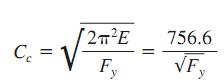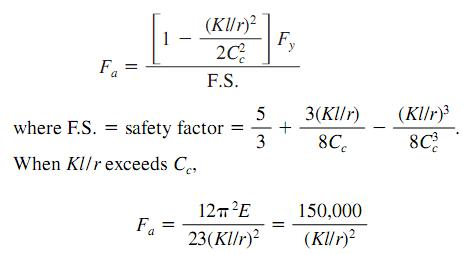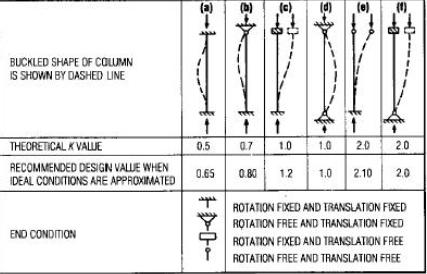Search

# Allowable Stress Design For Building Columns

Posted in Building |Email This Post |

The AISC specification for allowable-stress design (ASD) for buildings provides two formulas for computing allowable compressive stress Fa, ksi (MPa), for main members. The formula to use depends on the relationship of the largest effective slenderness ratio Kl/r of the cross section of any unbraced segment to a factor C c defined by the following equation and table below:Where
E=modulus of elasticity of steel =29,000 ksi ( 128.99 GPa )
Fy=Yield stress of steel, ksi (MPa)

When Kl/r is less than C c ,
Fy=36, Cc =126.1
Fy=50, Cc =107The effective-length factor K, equal to the ratio of effective-column length to actual unbraced length, may be greater or less than 1.0. Theoretical K values for six idealized conditions, in which joint rotation and translation are either fully realized or nonexistent, are tabulated in Figure belowMore Entries :